# SSAT Middle Level Math : How to find the common part with a Venn Diagram

## Example Questions

← Previous 1

### Example Question #1 : How To Find The Common Part With A Venn Diagram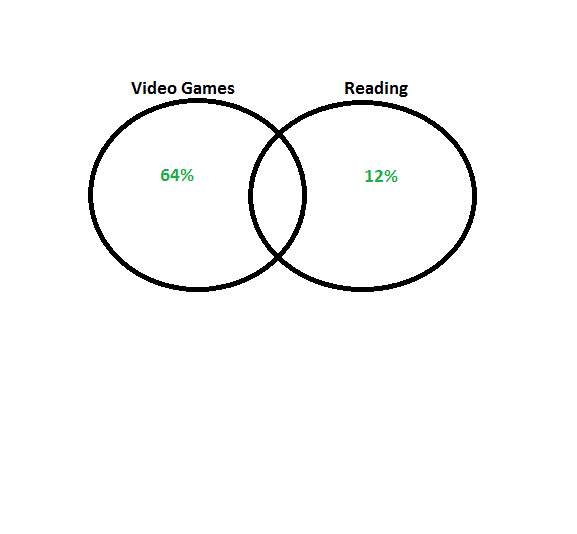Using the Venn diagram shown above, what fraction represents the amount of students that enjoy both reading and playing video games?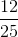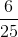Explanation:

The total number of survey respondents is equal topercent. Since the common part of the Venn diagram represents the respondents that enjoy both video games and reading, the solution is: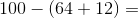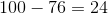Thus,percent is equal to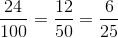### Example Question #2 : How To Find The Common Part With A Venn DiagramThe Venn diagram above, shows the results from a recent survey. The respondents were asked whether they enjoy playing video games, reading, or both. What percentage of the survey respondents enjoy both video games and reading?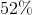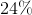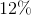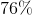Explanation:

The total number of survey respondents is equal topercent.

Since the common part of the Venn diagram represents the respondents that enjoy both video games and reading, the solution is:### Example Question #3 : How To Find The Common Part With A Venn Diagram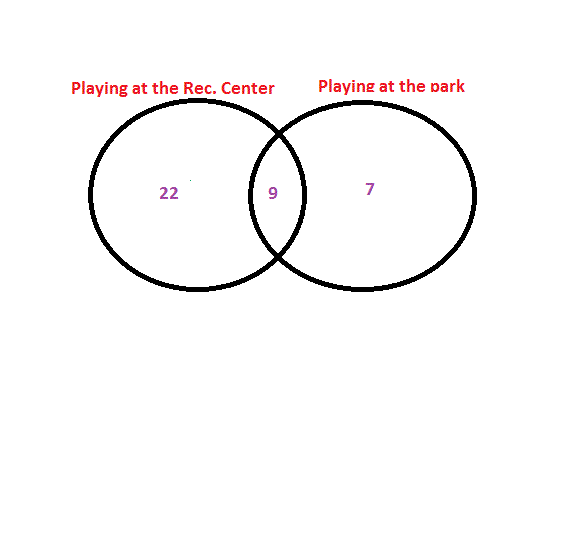The above Venn diagram depicts the number of students that: only play at the Rec. Center after school, only play at the park after school, and the students that play at both locations after school.

How many students play at both the park and Rec. Center after school?Explanation:

To solve this problem, locate the common part of this Venn diagram. Since each of the two outside portions of the Venn diagram represent students that only play at either the park or Rec. Center.

Thestudents in the common area of the Venn diagram, play both at the park and at the Rec. Center.

### Example Question #4 : How To Find The Common Part With A Venn DiagramUsing the Venn diagram above, what fraction of the respondents play at both the Rec. Center and the park. (Keep in mind that the two outside portions of the Venn diagram represent respondents that only play at one location or the other).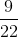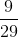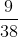Explanation:

To solve this problem, locate the common part of this Venn diagram. Since each of the two outside portions of the Venn diagram represent students that only play at either the park or Rec. Center. Thestudents in the common area of the Venn diagram belong to the group that plays at both the park and the Rec. Center.

This means that the total number of students (the denominator) must equal: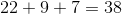Makingthe correct answer.

### Example Question #1 : How To Find The Common Part With A Venn Diagram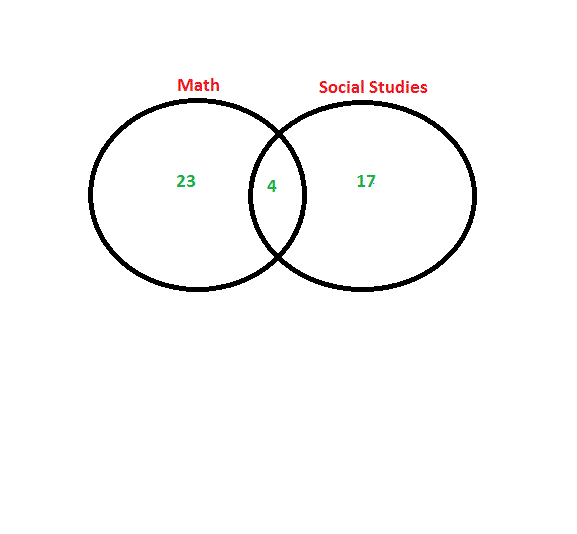The Venn diagram shown above represents the number of students that: only took math during summer school, only took social studies during summer school, and the number of students that took both math and social studies during summer school.

How many students took both math and social studies?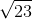Explanation:

The common portion of this Venn diagram is equal to, which depicts the number of students that took both math and social studies during summer school.

### Example Question #6 : How To Find The Common Part With A Venn DiagramWhat fraction of the above Venn diagram represents the number of students that took both math and social studies during summer school?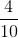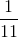Explanation:

The common portion of this Venn diagram is equal to, which depicts the number of students that took both math and social studies during summer school.

The denominator of the fraction is equal to the total number of students: \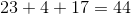Thus,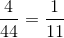### Example Question #7 : How To Find The Common Part With A Venn Diagram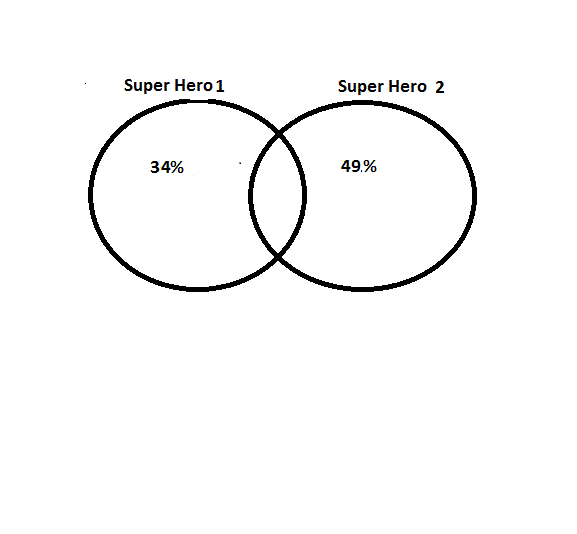Using the Venn diagram above shows whether respondents only like Super Hero, only like Super Hero, or if they like both Super Heroes. What percentage of respondents like both Super Heroes?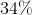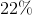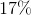Explanation:

The total number of survey respondents is equal topercent. Since the common part of the Venn diagram represents the respondents that like both Super Heroes.

Thus, the solution is: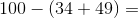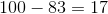### Example Question #1 : How To Find The Common Part With A Venn Diagram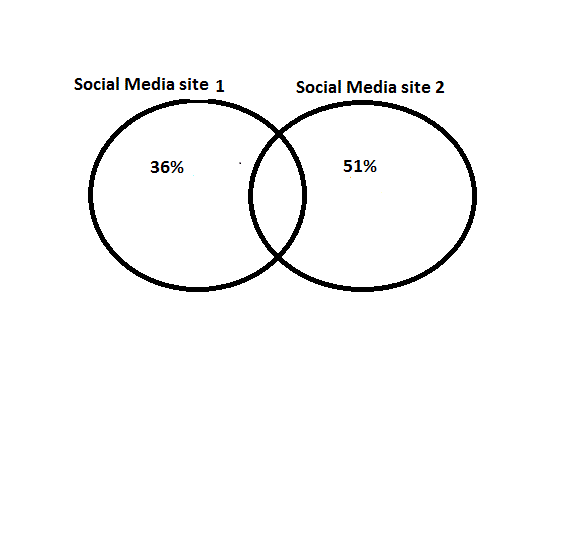The Venn diagram shown above displays whether respondents only use Social Media site, only use Social Media site, or use both of the Social Media sites.

What percent of respondents use both Social Media sites?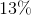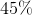Explanation:

The total number of survey respondents is equal topercent. Since the common part of the Venn diagram represents the respondents that use both of the Social Media sites, the solution is: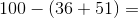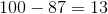### Example Question #2 : How To Find The Common Part With A Venn DiagramWhat fraction represents the amount of respondents that use both Social Media siteand Social Media site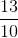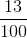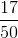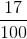Explanation:

The total number of survey respondents is equal topercent. Since the common part of the Venn diagram represents the respondents that use both social media sites, the solution is: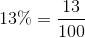### Example Question #1 : Venn Diagrams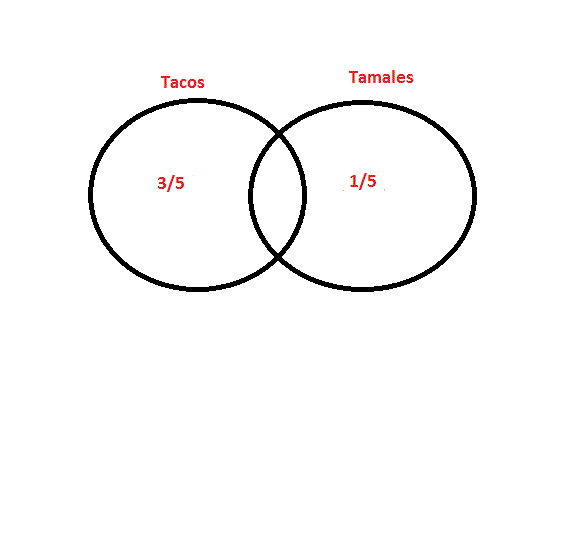The above Venn diagram shows the amount of survey respondents that only like tacos, only like tamales, and those that like both tacos and tamales.

What fraction of respondents like both tacos and tamales?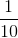Explanation:

The total number of survey respondents is equal topercent, which equals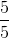The total number of respondents that do not like both tacos and tamales: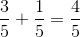.

The remaining amount equals the respondents that like both tacos and tamales.

Thus, the solution is: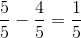← Previous 1